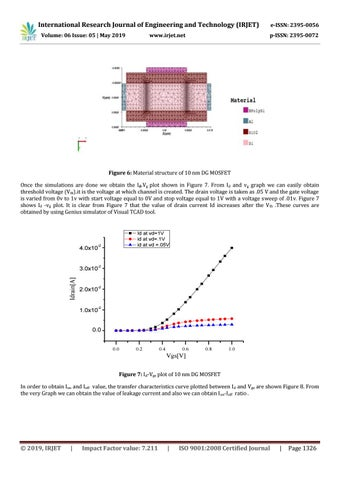International Research Journal of Engineering and Technology (IRJET) Volume: 06 Issue: 05 | May 2019

www.irjet.net

e-ISSN: 2395-0056 p-ISSN: 2395-0072

Figure 6: Material structure of 10 nm DG MOSFET

Idrain[A]

Once the simulations are done we obtain the Id-Vg plot shown in Figure 7. From Id and vg graph we can easily obtain threshold voltage (Vth).it is the voltage at which channel is created. The drain voltage is taken as .05 V and the gate voltage is varied from 0v to 1v with start voltage equal to 0V and stop voltage equal to 1V with a voltage sweep of .01v. Figure 7 shows Id -vg plot. It is clear from Figure 7 that the value of drain current Id increases after the V th .These curves are obtained by using Genius simulator of Visual TCAD tool.

4.0x10

-2

3.0x10

-2

2.0x10

-2

1.0x10

-2

Id at vd=1V id at vd=.1V id at vd =.05V

0.0

0.0

0.2

0.4

0.6

0.8

1.0

Vgs[V] Figure 7: Id-Vgs plot of 10 nm DG MOSFET In order to obtain Ion and Ioff value, the transfer characteristics curve plotted between I d and Vgs are shown Figure 8. From the very Graph we can obtain the value of leakage current and also we can obtain I on-Ioff ratio .

ÂŠ 2019, IRJET

|

Impact Factor value: 7.211

|

ISO 9001:2008 Certified Journal

|

Page 1326

IRJET- Simulation of 10nm Double Gate MOSFET using Visual TCAD Tool

https://www.irjet.net/archives/V6/i5/IRJET-V6I5267.pdf

IRJET- Simulation of 10nm Double Gate MOSFET using Visual TCAD Tool

https://www.irjet.net/archives/V6/i5/IRJET-V6I5267.pdf